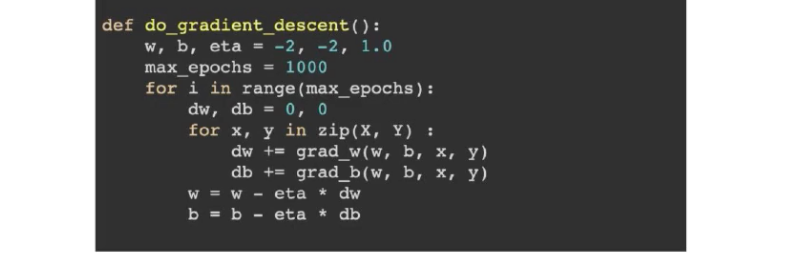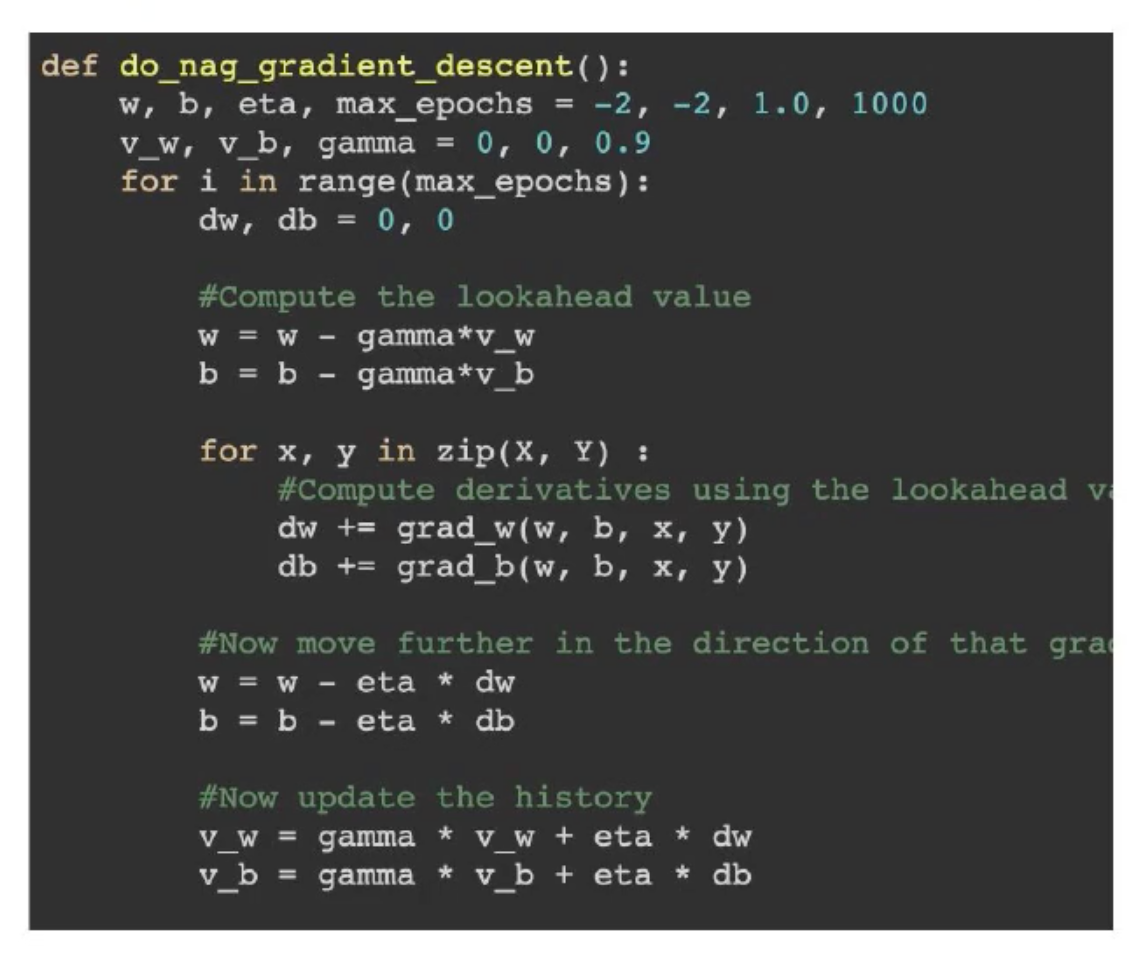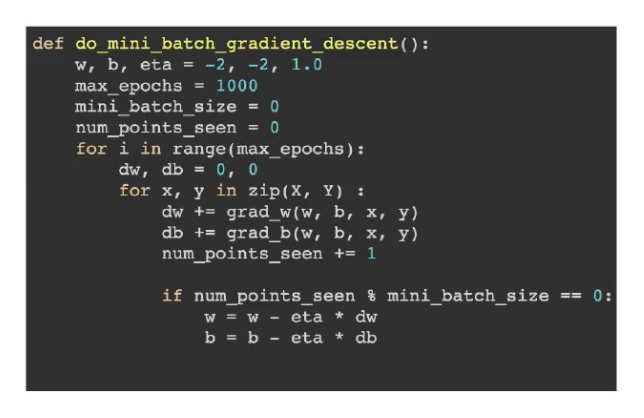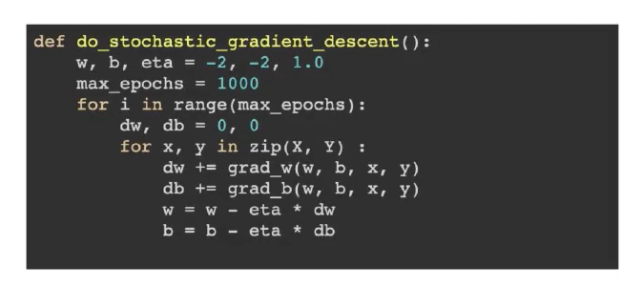# More on Gradient Descent Algorithm and other effective learning Algorithms…

A formal introduction with the mathematical derivation of the gradient descent algorithm is given in my last post on Sigmoid Neuron (Logistic Regression). But gradient descent update rule has its own limitations of slow convergence on a few scenarios. For example, when ‘w’ and ‘b’ are initialized on the high loss plateau point (as shown in the below picture highlighted as 1), the rate of change of ‘w’ and ‘b’ is minimum. The rate of change of ‘w’ and ‘b’ increases as the slope increases(highlighted as 2 in the below picture). Hence the number of epochs required will be extremely high, based on the initialization of ‘w’ and ‘b’.

Another limitation of gradient descent update rule is that the learning can stop if the points are stuck in the local minima. Please refer to the adjacent picture, where, based on the random initiation, ‘w’ and ‘b’ learning gets saturated with the local minima, and even after 1000 epochs the algorithm can not converge to the global minima.

To address the above 2 disadvantages of the gradient descent update rule, let us consider two main questions to be answered.

“What data should be used to compute the gradients?”

“How do we compute the gradients?”

In order to answer the above two questions, I introduce 3 ways data can be used to compute the gradients and 2 other ways to compute the gradients (apart from Gradient Descent). We can make a combination of these ways to generate accurate results.

#### Need for a better algorithm:

The following issue in gradient descent update rule motivates to go for a better algorithm.

Flatter error surface causes very very small gradients, in turn taking numerous epochs for negligible movement of ‘w’ and ‘b’.

### Momentum-based GD:

The above issue led to the birth of momentum based gradient descent update rule, where, ‘w’ and ‘b’ are updated not just based on the current updates(derivative), but also the past updates(derivatives). In the equation on the left, ‘gamma * vt-1’ represents the history component.

With the history component, during every timestep, the gradient moves the current direction as well as the previous gradient direction, where ‘gamma’ value ranges from 0 to 1. Hence momentum based gradient descent update rule takes the exponentially decaying averages of the gradient.Code snippet for implementing momentum based gradient descent update rule

#### The disadvantage of momentum-based GD:

Momentum-based GD is able to take larger steps even in the regions of the gentle slopes, as the momentum of the history gradients is carried with it every step. But is taking a larger step is always better? How about when the global minimum is about to be reached? Would there be a situation where momentum would cause us to run past our goal? Consider the below example where the momentum-based GD overshoots and moves past the global minimum.

Although Momentum-based GD is faster than GD, it oscillates in and out of the minima valley. But can this oscillation be reduced? Of course, Nesterov Accelerated GD helps us to reduce the oscillations that happen in momentum-based GD.

### Nesterov Accelerated GD:

We can notice in the above formulae for Momentum-based GD that it comprises of two components. One is the current derivative and the other is the history component. This implies the movement is multiplied., one based on history and again based on the current gradient. So if anyway the movement is going to be multiplied, why not move the history component first, and then calculate the derivative and update later, as specified in the picture above.Nesterov Accelerated GD

The red curve in the below picture shows ‘w’ and ‘b’ movement for Nesterov Accelerated GD. We can clearly see the oscillations are reduced when compared to the red curve of Momentum-based GD update rule.

### HOW DO WE COMPUTE THE GRADIENTS?

Knowing better algorithm that GD, now let us try to understand how to compute the gradients? Whether to use complete data or to use them partially? If partial, how? How many updates do we make?Code for batch GD

Generically, we make one update after the gradient is calculated for all the data points in one epoch. That means the update is done epoch number of times. We do this because our loss function is the sum over the loss at all the data points. Hence the derivate is the sum over the partial derivate of all the data points for one epoch. This is batch GD. So batch GD consults all the data points before making an update to the loss function. Ideally, in high-dimensional datasets, update becomes CPU intensive process. To handle such situations, we have options for Stochastic and mini-batch GD.Code for mini-batch GD

If we can compute the derivates for one batch of data points (say, 10 or 20) and update the parameters, that is mini-batch GD. This will help us to move to the global minima quick considering the high dimensional datasets. Mini-batch GD has many advantages over Stochastic and batch GD.Code for Stochastic GD

If the update can be done for every data point, then that is Stochastic GD. So, we make an update to the loss function for every data points. Stochastic GD has the advantage of making frequent and quicker updates in one epoch but has a disadvantage of approximating the gradient.

Depending on the case, batch, mini-batch or Stochastic GD can be used. But generally, mini-batch GD is used commonly.

### Better Algorithm — for adaptive learning rate:

Why do we need an adaptive learning rate? In most of the cases, datasets are sparse. And clearly derivative of the loss function with respect the weight is proportional to the corresponding input (x1 and x2 are the inputs).

When the input is sparse, most of the input value is 0. When the input value is zero, the derivative of the loss function with respect to the weight is 0, hence the update is 0. If the update is not happening, then there is a problem in converging to the global minima. So whenever the update happens, it should happen at a greater rate. Hence we need a greater learning rate whenever the update is non-zero. In other words, the learning rate should be adaptive to the feature that we deal with. If we are dealing with a sparse feature, the learning rate should be high and if we are dealing with dense features, the learning rate should be very less.

Precisely, we need a different learning rate for each parameter which takes care of the frequency of the features.

#### AdaGrad:

In AdaGrad we decay the learning rate for parameters in proportion to the update history (fewer the updates, lesser the decay). On the left is the formula for Adagrad. When Vt is larger (for datasets with very frequent updates), the effective learning rate is smaller. And when Vt is smaller (in case of sparse features), the effective learning rate is higher. AdaGrad has an advantage that parameters corresponding to the sparse features get better, but with respect to dense features, the learning rate decays rapidly and becomes zero and the update never happens. This is an issue with AdaGrad.

#### RMSProp:

Why not the decay of the denominator be avoided? RMSProp helps to achieve this by introducing another hyperparameter.

#### ADAM:

ADAM combines the advantages of momentum-based GD update rule and RMSProp. ADAM uses a cumulative history of gradients. It maintains one history, which is the running sum of all the updates (used to make update) and another history to adjust the learning rate. Added to this, ADAM also does Bias correction.

In Practice, ADAM with mini-batch of sizes(32,64,128) is the most preferred combination.

Thank you for reading my post. My next post will be on initialization methods and activation functions. Stay tuned.